Function Repository Resource:

# PixelSort

Sort pixels of an image to generate a pixel sorting glitch effect

Contributed by: Christian Pasquel
 ResourceFunction["PixelSort"][img] applies a pixel sorting effect to img. ResourceFunction["PixelSort"][img,assoc] applies a pixel sorting effect using the parameters specified in the association assoc.

## Details

This function is based on the ASDF Pixel Sort Processing script developed by Kim Asendorf (2010).
The algorithm first computes an image based on the specified provided property (e.g. "Luminance") and sorts its pixels. The sorted pixels are mapped to the original image to produce its pixel sorted version. The intermediate image computed using the specified property, with its sorted pixels, can be returned instead of the original image by setting the "OriginalImage" component of the association assoc to False.
The pixel sorting algorithm parameters can be specified by giving the following components in the association assoc:
 "Direction" "Row" direction in which the sorting is applied ("Row" or "Column") "Property" "Luminance" computed pixel property to sort (mode) "Level" 0.2 filter level (real 0 through 1) "LevelTest" "Min" whether the specified filter level is a maximum or a minimum "Reversed" False True to sort the pixels into reverse canonical order "OriginalImage" True False to return the image computed using the specified property (e.g. "Luminance") with its pixels sorted
Possible values for "Property" include:
 "Luminance" computes the pixels' RGB luminance by using the formula {r*0.2126,g*0.7152,b*0.0722} "Brightness" computes the pixels' RGB brightness by taking the mean of the values of the pixels
Possible values for "LevelTest" include:
 "Min" use the specified level as a minimum when filtering pixels "Max" use the specified level as a maximum when filtering pixels

## Examples

### Basic Examples (3)

Get a sample image:

 In:=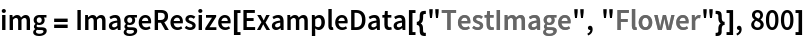Out=Sort the image pixels using the default values:

 In:=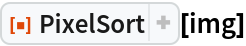Out=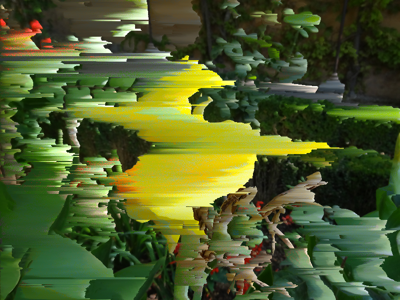Sort the image pixels using custom values:

 In:=Out=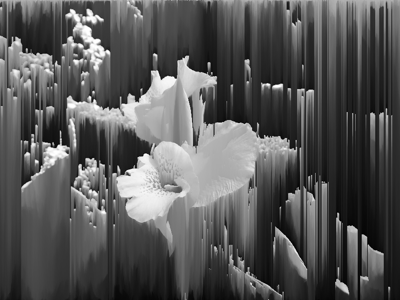### Scope (11)

Get some sample images:

 In:=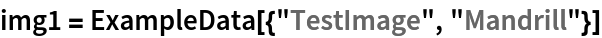Out=In:=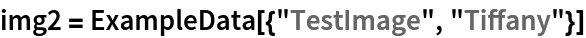Out=In:=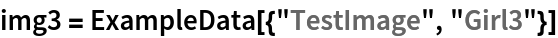Out=Sort rows of pixels of an image:

 In:=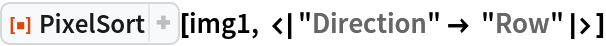Out=Sort columns of pixels of an image:

 In:=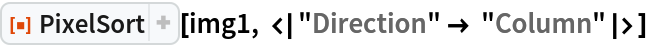Out=Sort the pixels of an image based on their luminance:

 In:=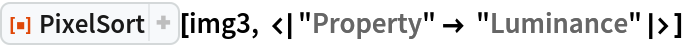Out=Sort the pixels based on their brightness:

 In:=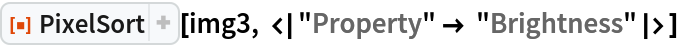Out=Use a custom filter level:

 In:=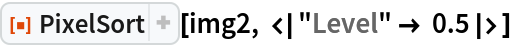Out=Sort all pixels with a minimum luminance value of 0.7:

 In:=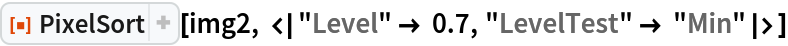Out=Sort all pixels with a maximum brightness value of 0.7:

 In:=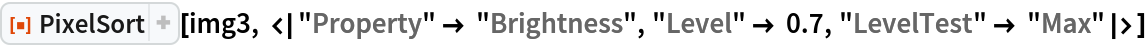Out=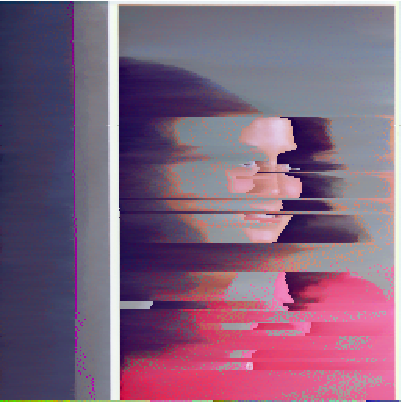Sort the pixels into reverse order:

 In:=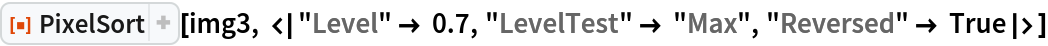Out=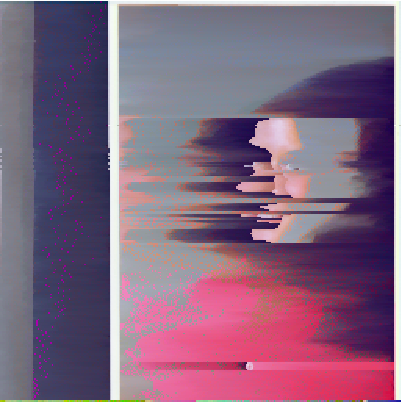Apply the filter on the image generated by computing the default luminance property:

 In:=Out=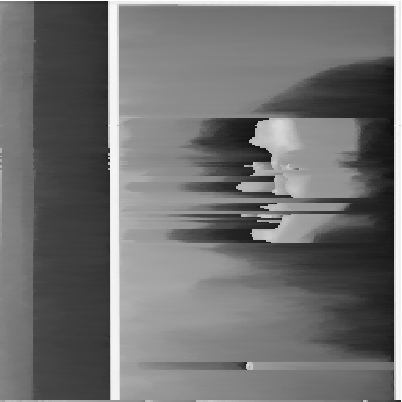Apply the filter on the image generated by computing the brightness of its pixels:

 In:=Out=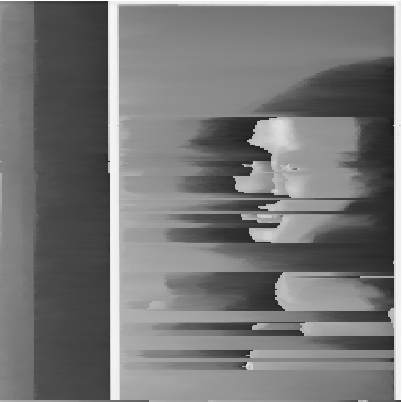### Properties and Relations (1)

There are subtle differences between using the "Luminance" and "Brightness" properties:

 In:=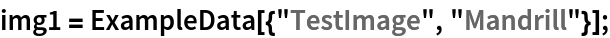In:=Out=### Possible Issues (2)

An invalid property will return an error:

 In:=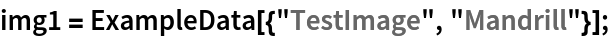In:=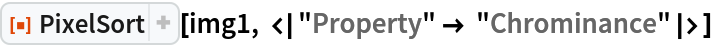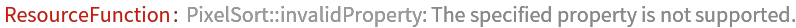Out=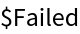An invalid level test will return an error:

 In:=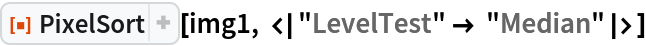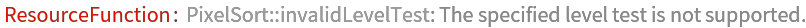Out=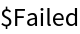### Neat Examples (2)

Get a test image:

 In:=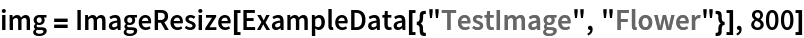Out=Create a pixel sorting animation:

 In:=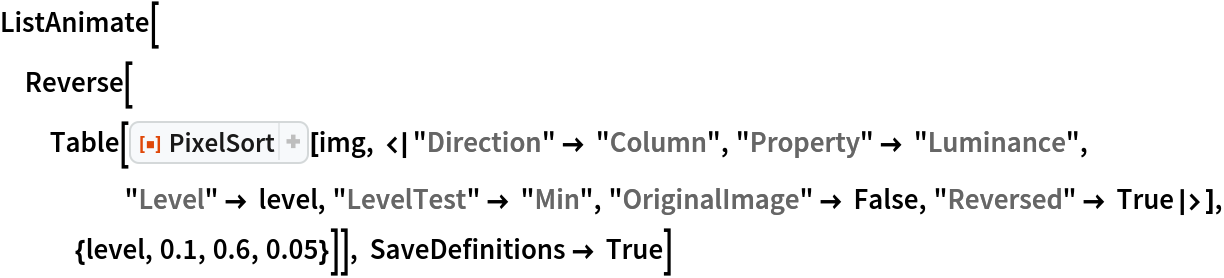Out=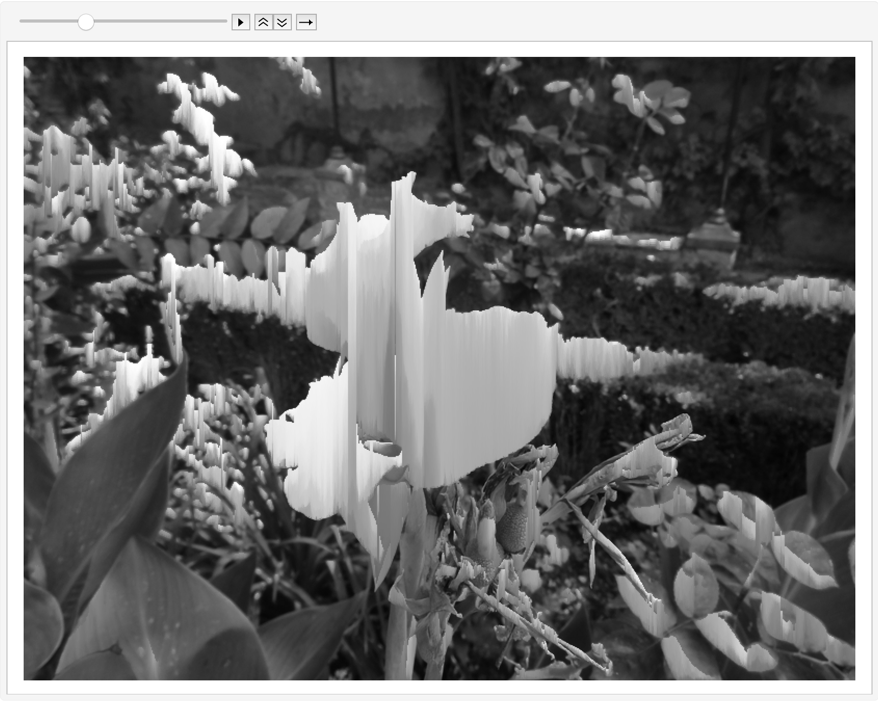## Version History

• 1.0.0 – 23 March 2021

## Author Notes

While this function is based on ASDF Pixel Sort by Kim Asendorf, it does not replicate the whole source code's algorithm. The original sorting modes (black (0), brightness (1), white(2)) have not been implemented as such. Instead, a luminance and a brightness modes are available (specified by using the "Property" component). Future updates of the function will include more modes and also the ability to provide a custom function as mode.